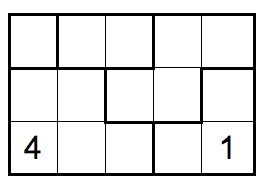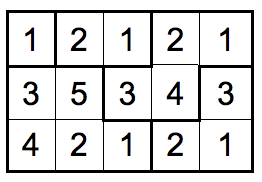시간 제한메모리 제한제출정답맞힌 사람정답 비율
2 초 512 MB42266.667%

## 문제

At some point or another, most computer science students have written a standard Sudoku solving program. This is yet another “put numbers in a grid’’ puzzle.

Numbers are placed in the grid so that each outlined region contains the numbers 1 to n, where n is the number of squares in the region. The same number can never touch itself, not even diagonally.Incomplete Grid Solution Grid

For this problem, you will write a program that takes as input an incomplete puzzle grid and outputs the puzzle solution grid.

## 입력

The first line of input contains a single decimal integer P, (1 ≤ P ≤ 100), which is the number of data sets that follow. Each data set should be processed identically and independently.

Each data set starts with a line containing the data set number K, (1 ≤ K ≤ 100), the number of rows in the input grid R, (1 ≤ R ≤ 7), and the number of columns in the input grid C, (1 ≤ C ≤ 7), separated by spaces. The next R lines contain a representation of the incomplete input grid, one row per line. The value in each cell is represented by either the digit already in that cell or a ‘-’ for an initially empty cell.

This grid is followed by a description of the separate regions in the grid. The first of these lines specifies the total number of regions. This is followed by one line for each region that specifies the cells contained in that region. Each region description consists of a decimal number N, specifying the number of cells in the region, followed by N cell descriptions separated by spaces. Each cell description consists of a left parenthesis, followed the cell’s row index, followed by a comma, followed by the cell’s row number, followed by a right parenthesis.

## 출력

For each data set, the output consists of the data set number, K, on a line by itself. This is followed by R lines containing C digits (separated by single spaces) showing the solution grid for the corresponding input data set.

## 예제 입력 1

2
1 3 5
- - - - -
- - - - -
4 - - - 1
5
1 (1,1)
2 (1,2) (1,3)
5 (2,1) (2,2) (3,1) (3,2) (3,3)
4 (2,3) (2,4) (1,4) (1,5)
3 (3,4) (3,5) (2,5)
2 7 7
- - - - - - -
4 - - - - 5 -
- - - - - - -
- - - 3 - - -
- - - - - - -
- - - - - - -
- - - - - - -
14
5 (1,1) (2,1) (3,1) (2,2) (2,3)
4 (2,4) (1,2) (1,3) (1,4)
2 (1,5) (1,6)
1 (1,7)
4 (4,1) (5,1) (6,1) (5,2)
1 (7,1)
4 (3,2) (4,2) (4,3) (5,3)
3 (7,3) (7,2) (6,2)
4 (6,3) (6,4) (7,4) (7,5)
6 (2,5) (3,5) (4,5) (3,6) (2,6) (2,7)
4 (3,7) (4,7) (4,6) (5,6)
1 (5,7)
5 (7,7) (7,6) (6,7) (6,6) (6,5)
5 (3,3) (3,4) (4,4) (5,4) (5,5)


## 예제 출력 1

1
1 2 1 2 1
3 5 3 4 3
4 2 1 2 1
2
3 1 4 2 1 2 1
4 2 5 3 6 5 4
1 3 1 4 2 3 1
2 4 2 3 1 4 2
1 3 1 5 2 3 1
4 2 4 3 4 5 2
1 3 1 2 1 3 1